Courses

# Networks & Control Systems - MCQ Test - 1

## 15 Questions MCQ Test GATE Electrical Engineering (EE) 2022 Mock Test Series | Networks & Control Systems - MCQ Test - 1

Description
This mock test of Networks & Control Systems - MCQ Test - 1 for GATE helps you for every GATE entrance exam. This contains 15 Multiple Choice Questions for GATE Networks & Control Systems - MCQ Test - 1 (mcq) to study with solutions a complete question bank. The solved questions answers in this Networks & Control Systems - MCQ Test - 1 quiz give you a good mix of easy questions and tough questions. GATE students definitely take this Networks & Control Systems - MCQ Test - 1 exercise for a better result in the exam. You can find other Networks & Control Systems - MCQ Test - 1 extra questions, long questions & short questions for GATE on EduRev as well by searching above.
QUESTION: 1

Solution:
QUESTION: 2

Solution:
QUESTION: 3

### The reactance offered by a capacitor to alternating current of frequency 50 Hz is 20 Q. If frequency is increased to 100 Hz, reactance becomes_____ohms.

Solution:
QUESTION: 4

Given the current through R is i = 1A. The value of R is ______________ Ω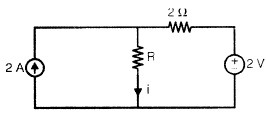Solution:
QUESTION: 5

A series RLC circuit has bandwidth as 1000 Hz with R = 10 Ω. If the resistance ‘R’ increased to 20 Ω, then the bandwidth of  the circuit is __________

Solution:
QUESTION: 6

In a series RL circuit, 12 V rms is measured across the resistor, and 14 V rms is measured across the inductor. The peak value of the source voltage is

Solution:
QUESTION: 7

Current in some branches are given in the below figure. The value of T is ____________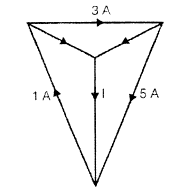Solution:
QUESTION: 8

The circuit shown below is the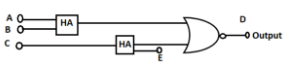Solution:
QUESTION: 9

A system has bandwidth as 4000 Hz with rise time 2.5 seconds. If the rise time decreased to 1.25 seconds then the bandwidth is ___________ Hz

Solution:
QUESTION: 10

A system with pole at -1 +j had settling time as 3 secs. If the pole shifted to -2+3j the settling time is _________ sec

Solution:
QUESTION: 11

In “force - current” analogy, equivalent of viscous coefficient is _________ and in “force - voltage" analogy, equivalent of spring constant is ______________

Solution:
QUESTION: 12

A system has transfer function (5 - S)/(5 + S). It is a _______

Solution:
QUESTION: 13

Consider the following ‘3’ transfer functions.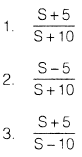Among these minimum phase system (s) i s/are

Solution:
QUESTION: 14

The purpose of Pi controller is to improve ___________ of the system.

Solution:
QUESTION: 15

Below figure is a pole-zero plot of a ____________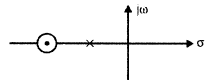Solution: